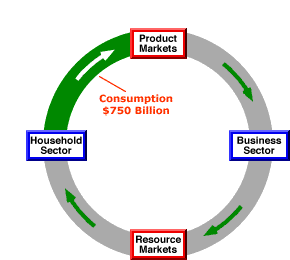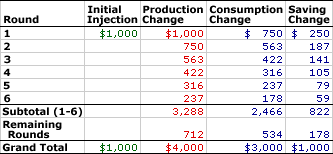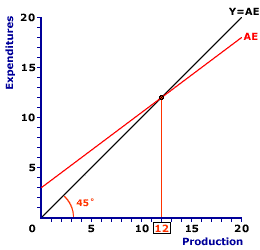Friday  February 3, 2023
 AmosWEB means Economics with a Touch of Whimsy!WEALTH DISTRIBUTION: The manner in which wealth is divided among the members of the economy. A perfectly equal wealth distribution would mean everyone in the country has exactly the same wealth. In reality, wealth is unequally distributed. A few people have a great deal of wealth and most others have less. Any well-functioning economy, that's doing a pretty good job of satisfying consumer wants and needs, will have some degree of inequality in the distribution of wealth. This occurs because some people have done a good job of producing what people want, and thus grow wealthy. However, wealth tends to perpetuate itself, over and above what may be justified by valuable production. Along with wealth comes market control, political power, and the ability to accumulate more wealth at the expense of others.MULTIPLIER PRINCIPLE:

The cumulatively reinforcing induced interaction between consumption, production, factor payments, and income that amplifies autonomous changes in investment, government spending, exports, taxes, or other shocks to the macroeconomy. The multiplier principle is so named because relatively small autonomous changes generate relatively larger, or multiple, induced changes in aggregate production. This principle is commonly represented by a multiplier, which is a specific number with a value greater than one.
The essence of the multiplier principle is that relatively small changes in autonomous expenditures or other shocks cause relatively large overall changes in aggregate production and income. The multiplier principle works because a change in autonomous expenditures triggers a change in aggregate production, factor payments, and income, which then induces changes in other expenditures, especially consumption. These induced expenditures then cause further changes in aggregate production, factor payments, and income, when then induce further changes in expenditures. The process is cumulative and reinforcing.

The multiplier principle is a direct implication of Keynesian economics. The key to the multiplier principle is induced expenditures, expenditures that depend on aggregate production and income, especially induced consumption expenditures. Induced consumption expenditures, as captured by the marginal propensity to consume, are the cornerstone of Keynesian economics.

### A Circular Flow Injection

The multiplier principle is best illustrated using the circular flow model of the economy. The circular flow is a model of the continuous production, factor payment, income, and expenditure interaction among the four major sectors (household, business, government, and foreign), that takes place through the three aggregated macroeconomic markets (product, resource, and financial).

The Circular FlowA simplified circular flow model is presented in the exhibit to the right. The four key parts of this circular flow are the business and household sectors at the right and left, and the product and resource markets at the top and bottom. This model can be used to illustrate the multiplier principle.

To get the flow moving, let's see what would happen with a \$1 trillion change in autonomous investment expenditures, a injection of investment into the circular flow. Click the [Inject Investment] button to illustrate this. What happens?

• First, \$1 trillion enters into the product markets for the purchase of \$1 trillion worth of capital goods.

• Second, this \$1 trillion is revenue received by the business sector as payment for this production.

• Third, this \$1 trillion revenue is then used by te business sector as factor payments to the resources that produce the capital goods.

• Fourth, this \$1 trillion of factor payments becomes income of the household sector.

• Fifth, the \$1 trillion of income received by the household sector is then divided between consumption (\$750 billion) and saving (\$250 billion), based on the marginal propensity to consume (and save).
Of some importance, this \$250 billion of saving is a leakage out of the circular flow. Also of some importance, the \$750 of consumption expenditures remains in the circular flow and is used to purchase additional production.

### Another Round

The \$750 of consumption expenditures remaining in the circular flow generates another round of aggregate production, factor payments, income, and consumption.

The Circular FlowThe new exhibit to the right presents the circular flow model as it stands with the \$750 billion of consumption expenditures poised to enter the production markets for the payment of aggregate production. Click the [Induced Consumption] button to illustrate the results of this additional round of expenditures.

While the numbers are a bit different, the process is much the same.

• First, \$750 billion enters into the product markets for the purchase of \$1 trillion worth of consumption goods.

• Second, this \$750 billion is once again revenue received by the business sector as payment for the production.

• Third, this \$750 billion revenue is then used by te business sector as factor payments to the resources that produce this round of consumption goods.

• Fourth, this \$750 billion of factor payments becomes additional income of the household sector.

• Fifth, the \$750 billion of income received by the household sector is once again divided between consumption (\$563 billion) and saving (\$187 billion), based on the marginal propensity to consume (and save).
With this round, a portion of the \$750 billion household sector is leaked out of the circular flow as saving and a portion remains as consumption expenditures, which will generate another round of aggregate production, factor payments, income, and then even more consumption expenditures. And so it goes.

### A Bunch of Rounds

The circular flow is likely to experience several additional rounds of aggregate production, factor payments, income, and consumption expenditures. Each subsequently round, however, is smaller than the previous round, meaning that the process eventually winds down to infinitely small values.

But with each round, additional aggregate production is generated. The first round generates \$1 trillion of capital goods production. The second round generates \$750 billion of consumption goods production. The third round adds another \$563 billion of consumption goods production to the total.

The first three rounds has a total of \$2.313 trillion, almost 2 1/2 times the initial injection of investment expenditures. How much higher will it go?

A Bunch of RoundsThe answer is given by the exhibit to the right, which summarizes several rounds of the circular flow.

• The first round generates \$1 trillion in production, \$750 billion in consumption, and \$250 billion in saving. This round is triggered by the initial \$1 trillion investment in capital.

• The second round generates \$750 billion in production, \$563 billion in consumption, and \$187 billion in saving. This round is triggered by the \$750 billion of consumption from the first round.

• The \$563 billion of consumption from the second round then generates \$563 billion in production, \$422 billion in consumption, and \$141 billion in saving in the third round.

• The values for production, consumption, and saving are also presented for rounds four through six. The first six rounds combine for \$3,288 billion in production, \$2,466 billion in consumption, and \$822 billion in saving.
In these first six rounds, the increase in aggregate production of \$3,288 billion generates an identical \$3,288 billion of income. This income is divided between consumption and saving based on the marginal propensity to consume (0.75) and the marginal propensity to save (0.25). The \$2,466 billion in consumption is 75% of the income (\$2,466 = \$3,288 x 0.75). The \$822 billion in saving is 25% of the income (\$822 = \$3,288 x 0.25).
• The totals for remaining rounds of activity beyond round six (seven through infinity) are also listed in the table. These rounds generate and additional \$712 billion in production, \$534 billion in consumption, and \$178 billion in saving. Once again the production (and income) total is divided between consumption and saving based on the marginal propensities to consume and save.

• The grand totals of all rounds are presented at the bottom of the table, which are \$4 trillion in production, \$3 trillion in consumption, and \$1 trillion in saving. As might be expected the grand total of production (and income) is also divided between consumption and saving based on the marginal propensities to consume and save.
The overall change in aggregate production (\$4 trillion) is a multiple of the initial change in investment that triggered the process (\$1 trillion). In this case the multiple is four times -- aggregate production increases by four times the increase in investment. The change in aggregate production and income is divided between consumption (\$3 trillion) and saving (\$1 trillion) based on the marginal propensity to consume (0.75) and marginal propensity to save (0.25). The overall change in saving (\$1 trillion) is exactly the same as the initial change in investment (\$1 trillion). Using other terminology, injections equal leakages.

### The Keynesian Cross

The Keynesian CrossThe multiplier principle is commonly illustrated as a shift of the aggregate expenditures line in the Keynesian model. The exhibit to the right presents a standard Keynesian cross. The existing equilibrium is \$12 trillion, given by the intersection of the aggregate expenditures line (AE) and the 45-degree line (Y = AE). This equilibrium, however, is about to change.

Suppose, for example, that autonomous investment expenditures increase by \$1 trillion, which is just the thing to shift the aggregate expenditures line and trigger the multiplier.

To display the shift of the aggregate expenditures line, click the [\$1 Trillion More] button. This reveals a new aggregate expenditures line that is \$1 trillion higher than the original line.

The new equilibrium is found at the intersection of the 45-degree line and the new aggregate expenditures line, which is \$16 trillion of aggregate production. The difference between the original equilibrium and the new equilibrium is \$4 trillion. This is four times the initial change in investment, which implies a multiplier of 4.

Let's examine this adjustment process a little more closely.

• The initial change in investment causes a vertical shift of the aggregate expenditures line that disrupts the existing equilibrium. In particular, aggregate expenditures exceed aggregate production, which creates an economy-side shortage in the product markets and causes a decrease in business inventories. Click the [Autonomous Investment] button to highlight this initial disequilibrium gap between point A and point B. The business sector responds to this shortage and decrease in business inventories by increasing production.

• This boost in production means an increase in income to the household sector. The household sector is induced by this additional income to increase consumption expenditures. Click the [Induced Consumption] button to highlight this adjustment. This is the movement along the aggregate expenditures line from point B to point C. Because consumption is the only induced expenditure in this particular model, the slope of the aggregate expenditures line is equal to the marginal propensity to consume. The movement along the aggregate expenditures line is what restores balance between aggregate expenditures and aggregate production.
The movement along the aggregate expenditures line not only restores equilibrium it also generates the multiplier process. Each change in aggregate production on the supply side of the economy induces a change in consumption and aggregate expenditures on the demand side. The process of closing one gap between production and expenditures ends up creating another gap.

For example, the initial investment creates a \$1 trillion imbalance between production and expenditures. This gap is closed with \$1 trillion of production. However, this production induces \$750 billion of consumption, which creates a new \$750 billion gap. Closing this gap with \$750 billion of production induces another \$563 billion in consumption, which creates another new gap.

Fortunately the gaps grow smaller until they are inconsequential and can be ignored. The multiplier process ends when these gaps become infinitesimally small.

### The Simple Expenditures Multiplier

The multiplier principle is commonly represented by the multiplier, a measure of this cumulatively reinforcing induced interaction that is greater than one. The simplest multiplier, which is often used to illustrate the basics of the multiplier process, is the simple expenditures multiplier.

The simple expenditures multiplier is the ratio of the change in aggregate production to an autonomous change in an aggregate expenditure when consumption is the only induced expenditure. This simple expenditures multiplier is typically used to analyze shocks caused by changes in investment expenditures.

This simplest version of the simple expenditures multiplier comes from the two-sector Keynesian model that has nothing but induced consumption from the household sector and autonomous investment from the business sector. However, while this simple expenditures multiplier is derived from the basic two-sector Keynesian model, it works equally well for other models as long as consumption is the only induced expenditure.

This version is as simple as it gets while capturing the fundamentals of the multiplier. Autonomous investment triggers the multiplier process and induced consumption provides the cumulatively reinforcing interaction between consumption, aggregate production, factor payments, and income.

The formula for this simple expenditures multiplier, m, is:

 m = 1(1 - MPC) = 1MPS
Where MPC is the marginal propensity to consume and MPS is the marginal propensity to save.

If, for example, the MPC is 0.75 (and the MPS is 0.25), then an autonomous \$1 trillion change in investment expenditures results in a change in aggregate production of \$4 trillion.

While the simple expenditures multiplier can be derived from the basic two-sector Keynesian multiplier, it also works for models with more sectors, as long as consumption is the only induced expenditure. If, for example, autonomous government purchases change by \$1 trillion, then the change in aggregate production is \$4 trillion, the same as with a \$1 trillion change in investment expenditures. Moreover, the same change in aggregate production is realized if autonomous exports or consumption expenditures change by \$1 trillion.

 <= MULTIPLIER, KEYNESIAN CROSS MULTIPLIER, SLOPE OF AGGREGATE EXPENDITURES LINE =>Recommended Citation:

MULTIPLIER PRINCIPLE, AmosWEB Encyclonomic WEB*pedia, http://www.AmosWEB.com, AmosWEB LLC, 2000-2023. [Accessed: February 3, 2023].

Check Out These Related Terms...

Or For A Little Background...

And For Further Study...
Search Again?GREEN LOGIGUIN[What's This?] Today, you are likely to spend a great deal of time at the confiscated property police auction hoping to buy either a desktop calendar with all federal and state holidays highlighted or a half-dozen helium filled balloons. Be on the lookout for telephone calls from long-lost relatives.Your Complete ScopeOn a typical day, the United States Mint produces over \$1 million worth of dimes."There is a way to look at the past. Don't hide from it. It will not catch you - if you don't repeat it."-- Pearl Bailey, Singer and ActressICCHInternational Commodities Clearing HouseA PEDestrian's Guide Xtra CreditTell us what you think about AmosWEB. Like what you see? Have suggestions for improvements? Let us know. Click the User Feedback link.| | | | | | | | | | |
| | | |

Thanks for visiting AmosWEB